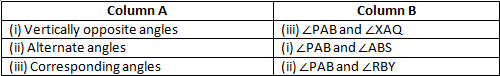# RD Sharma Solutions Class 6 Maths Chapter 15 Pair of Lines and Transversal

Read RD Sharma Solutions Class 6 Maths Chapter 15 Pair of Lines and Transversal below, students should study RD Sharma class 6 Mathematics available on Studiestoday.com with solved questions and answers. These chapter wise answers for class 6 Mathematics have been prepared by teacher of Grade 6. These RD Sharma class 6 Solutions have been designed as per the latest NCERT syllabus for class 6 and if practiced thoroughly can help you to score good marks in standard 6 Mathematics class tests and examinations

Exercise 15.1

Question 1: Identify parallel line segments shown in Fig. 15.6.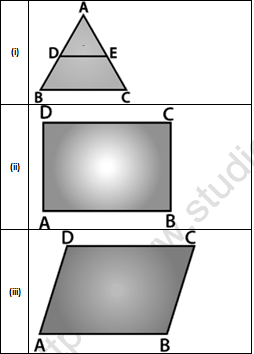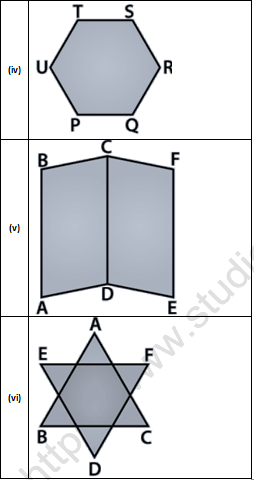Solution 1:
(i) By the given figure we can find that BC || DE.
(ii) By the given figure we can find that AB || DC and AD || BC.
(iii) By the given figure we can find that AB || DC and AD || BC.
(iv) By the given figure we can find that PQ || TS,UT || QR and UP || SR.
(v) By the given figure we can find that AB || EF || CD,BC || AD and CF || DE.
(vi) By the given figure we can find that EF || BC,AB || DF and AC || DE.

Question 2:   Name the pairs of all possible parallel edges of the pencil box whose figure is shown in Fig. 15.7.Solution 2:   The parallel edges of the pencil box are:-
AB || DC || HE || GF
AD || GH || BC || EF
AH || DG || BE || CF

Question 3:   In Fig. 15.8, do the segments AB and CD intersect? Are they parallel? Give reasons.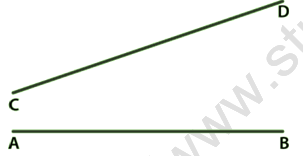Solution  3:   No, AB and CD line not intersect. But if we increase line CD from point C than it can intersect line AB.  AB and CD are not parallel lines because the distance between them is not same.

Question 4:   State which of the following statements are true (T) or which are false (F):
(i) If two lines in the same plane do not intersect, then they must be parallel.
(ii) Distance between two parallel lines is not same everywhere.
(iii) If m ⊥ l, n ⊥ l and m ≠ n, then m || n.
(iv) Two non-intersecting coplanar rays are parallel.
(v) If ray AB || line m, then line segment AB.
(vi) If line AB || line m, then line segment AB || m.
(vii) No two parallel line segments intersect.
(viii) Every pair of lines is a pair of coplanar lines.
(ix) Two lines perpendicular to the same line are parallel.
(x) A line perpendicular to one of two parallel lines is perpendicular to the other.

Solution 4:

(i) If two lines in the same plane do not intersect, then they must be parallel. True
(ii) Distance between two parallel lines is not same everywhere. False
(iii) If m ⊥ l, n ⊥ l and m ≠ n, then m || n. True
(iv) Two non-intersecting coplanar rays are parallel. False
(v) If ray AB || line m, then line segment AB. True
(vi) If line AB || line m, then line segment AB || m. True
(vii) No two parallel line segments intersect. True
(viii) Every pair of lines is a pair of coplanar lines. False
(ix) Two lines perpendicular to the same line are parallel. True
(x) A line perpendicular to one of two parallel lines is perpendicular to the other. True

Exercise 15.2

Question 1:   In Fig. 15.17, line n is a transversal to lines l and m. Identify the following: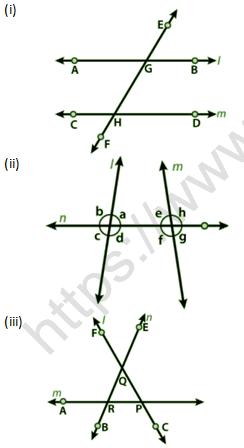(i) Alternate and corresponding angles in Fig. 15.17 (i).
(ii) Angles alternate to ∠d and ∠g and angles corresponding to ∠f and ∠h in Fig. 15.17 (ii).
(iii) Angle alternative to ∠PQR, angle corresponding to ∠RQF and angle alternate to ∠PQE in Fig. 15.17.
(iv) Pairs of interior and exterior angles on the same side of the transversal in Fig. 15.17 (ii).

Solution 1:

(i) The Alternate and corresponding angles according to given figure are below:-
Alternate interior Angles:- ∠BGH and ∠CHG; ∠AGH and ∠CHF
Alternate exterior Angles:- ∠AGE and ∠DHF; ∠EGB and ∠CHF
Corresponding Angles:- ∠EGB and ∠GHD; ∠EGA and ∠GHC; ∠BGH and ∠DHF; ∠AGF and ∠CHF.

(ii) The Alternate and corresponding angles according to given figure are below:-
Alternate Angles:-  ∠d and ∠g are ∠e and ∠b
Corresponding Angles :- ∠f and ∠h are ∠c and ∠a.

(iii) The Alternate and corresponding angles according to l is transversal to m and n:-
Alternate Angle:- ∠PQR is ∠QRA
Corresponding Angle:- ∠RQF is ∠BRA
Alternate Angle:- ∠PQE is ∠BRA

(iv) The Interior and Exterior angles according to given figure are below:-
Interior Angles:- ∠d, ∠f and ∠a, ∠e
Exterior Angles:- ∠c, ∠g and ∠b, ∠h

Question 2:  Match column A and column B with the help of the Fig. 15.18: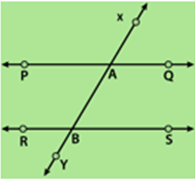Solution 2: